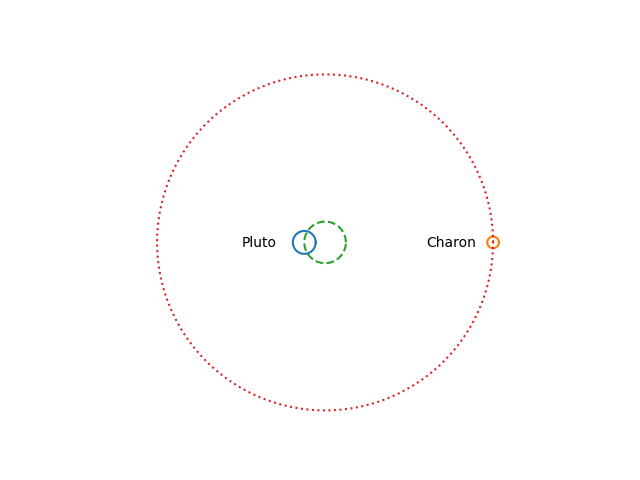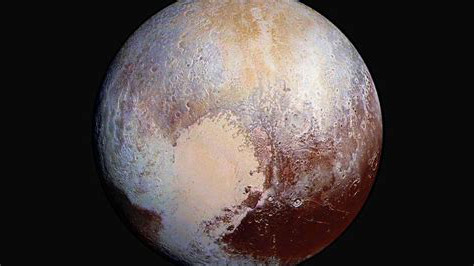# The Pluto-Charon orbit

The Moon doesn’t orbit the center of the Earth; it orbits the center of mass of the Earth-Moon system, which is inside the Earth. The distinction matters for designing satellite orbits, but it cannot be seen on a plot to scale. We’ll quantify this below.

Pluto’s moon Charon, however, is so large relative to Pluto and so close, that the center of mass of the Pluto-Charon system is outside of Pluto, and you can easily see this in a plot.Imagine Pluto and Charon sitting on each end of a balanced seesaw. Pluto is a distance x1 to the left of the fulcrum, and Charon is a distance x2 to the right of the fulcrum. Let m1 be the mass of Pluto and m2 be the mass of Charon. Then

m1 x1 = m2 x2

and

x1 = m2 (x1 + x2) / (m1 + m2).

Now let’s put in some numbers.

m1 = 1.309 × 1022 kg
m2 = 1.62 × 1021 kg
x1 + x2 = 19,640 km

From this we find

x1 = (1.62 × 19640 / 14.71) km = 2163 km

and so the distance from the center of Pluto to the center of mass of the Pluto-Charon system is 2163 km. But the radius of Pluto is only 1190 km. So the center of mass of the Pluto-Charon system is about as far above the surface of Pluto as the center of Pluto is below the surface.

## Comparison with the Earth-Moon system

It matters that the moon doesn’t exactly orbit the center of the Earth, but the difference between the center of the Earth and the center of mass of the Earth-Moon system is less dramatic. Let’s put in the numbers for the Earth and Moon.

m1 = 5.97 × 1024 kg
m2 = 7.346 × 1022 kg
x1 + x2 = 392,600 km

From this we find

x1 = (7.346 × 392,600 / 604) km = 4,775 km

The radius of Earth is 6,371 km, and so the center of mass of the Earth-Moon system is inside the Earth.

I made a plot analogous to the one above but for the Earth-Moon system. You could barely see the moon because it is so small relative to the size of its orbit. And you cannot see the difference between the center of the Earth and the barycenter of the Earth and Moon.

## Tidal locking

Not only is Charon tidally locked with Pluto, as our moon is with Earth, but Pluto is tidally locked with Charon as well.

On Earth we only ever see one side of the moon. We never see the “dark side,” which is more accurately the “far side.” But someone standing on the moon would see Earth rotate.

Someone standing on Pluto would only ever see one side of Charon, and someone standing on Charon would only ever see one side of Pluto. Sputnik Planitia, the big heart-shaped feature on Pluto, is on the opposite side of Charon, so you could say Pluto is hiding its heart from its companion.## One thought on “The Pluto-Charon orbit”

1. Jennifer German, NASA/JPL Solar System Ambassador

This is exactly what I needed, thank you! If I end up presenting on Pluto and Charon, may I use your plot of their orbits? I will of course credit you.